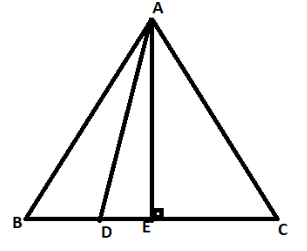QUESTION

# In an equilateral triangle $ABC$, $D$ is a point on side $BC$ such that $4BD = BC$. Prove that $16A{D^2} = 13B{C^2}$.

Hint: In this question draw a line perpendicular to the base of the triangle and use the property of an equilateral triangle in which perpendicular drawn from vertex to base bisects the base.Given
$ABC$ an equilateral triangle in which $4BD = BC$
$\therefore BD = \dfrac{{BC}}{4}$
Now draw a line $AE$ perpendicular to base $BC$
And as we know that in an equilateral triangle perpendicular drawn from vertex to base bisects the base.
Since $AE$ is perpendicular to $BC$ then we can say that:
$BE = EC = \dfrac{{BC}}{2}$
Now take triangle AED, apply the Pythagoras theorem we get:
$A{D^2} = A{E^2} + D{E^2}$.....take this as equation first
Similarly in triangle AEB
$A{B^2} = A{E^2} + B{E^2}$.....take this as equation second
Now putting the value of $A{E^2}$ from equation second in equation first we get:
$A{D^2} = A{B^2} - B{E^2} + D{E^2}$
Now, $AB = BC$as ABC is an equilateral triangle, $BE = \dfrac{{BC}}{2}$ proved above, $DE = BE - BD$ and $BD = \dfrac{{BC}}{4}$ given in question.
After replacing the values equation will be:
$\ A{D^2} = B{C^2} - {\left( {\dfrac{{BC}}{2}} \right)^2} + {\left( {BE - BD} \right)^2} \\ A{D^2} = B{C^2} - \dfrac{{B{C^2}}}{4} + {\left( {\dfrac{{BC}}{2} - \dfrac{{BC}}{4}} \right)^2} \\ A{D^2} = B{C^2} - \dfrac{{B{C^2}}}{4} + \dfrac{{B{C^2}}}{{16}} \\ A{D^2} = \dfrac{{16B{C^2} - 4B{C^2} + B{C^2}}}{{16}} \\ A{D^2} = \dfrac{{13B{C^2}}}{{16}} \\ 16A{D^2} = 13B{C^2} \\$
Hence proved $16A{D^2} = 13B{C^2}$

Note: In this question it's given that $4BD = BC$, from here we related that $BD = \dfrac{{BC}}{4}$ after that we applied the Pythagoras theorem in triangles AED and AEB and put the value of $A{E^2}$ from equation second in equation first, after that we replaced the values of $AB$, $BE$, $DE$ and $BD$ and further simplified the equation and got the final result as $16A{D^2} = 13B{C^2}$ which was required to be proved.